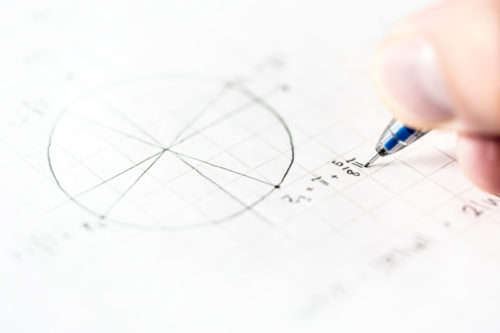# Upper Secondary A Math Tuition

## A Math Tuition For Secondary 3 and 4

Our Secondary 3 and Secondary 4 A Math tuition classes are tailored to our students’ needs and their learning styles. Our Secondary 3 A Math students have all developed a stronger foundation in dealing with the A Math syllabus and have thus gained a higher confidence when they move to Secondary 4. Getting extra help during Secondary 3 for the A Math subject especially if your child is not as confident in certain areas will really help to accelerate your child’s learning so that when he or she reaches Secondary 4, your child will be able to keep up with the syllabus taught in school.

### Amath##### Indices and Surds
• Apply algebra concepts to simplify indices expressions and express values in surds form.
• Solve indices equation and surds equations step by step using the most effective method.

##### Roots of Quadratic Equations and Discriminants
• Memorise and  identities and formulas for sum of roots, product of roots and the discriminants.  Apply the formulas correctly onto basic questions.
• Recognise the different forms of quadratic inequality and use the right method to solve.
• Understand the deeper concepts and master the various methods to solve common exam questions with all the steps clearly shown.

##### Remainder and Factor Theorem
• Solve different types of identities effectively by identifying the correct method to apply.
• To find the remainder when the original expression is divided by a linear factor or a quadratic factor.
• Solve algebra equations of higher orders of x (and above) by using factor theorem.  Factorise the expression using long division accurately.
• Compare 2 equations and observe a link between them and identify the correct transformation that will map 1 equation onto another equation by replacing x with another variable.

##### Trigo and Further Trigo
• Memorise the values of the trigo for special angles (both radian and degree) in surds form.
• Identify the quadrants correctly and solve trigo equations to work out all the angles within the given range.
• Prove trigo identities using the correct formulas.
• Sketch trigo curves accurately by working out the amplitude and period of 1 cycle.
• Solve further trigo problem sums using the R-formula.

##### Coordinates Geometry and Circles
• Find equations of straight lines including parallel lines, perpendicular lines and perpendicular bisectors using gradient and a pair of coordinates.
• Calculate the area of triangle or quadrilateral using the shoe-lace method.
• Draw out the perpendicular distance from a point to a line and formulate an equation using the area of triangle to solve for the perpendicular distance.
• Use ratios to find the coordinates of a point.
• Identify the radius and centre to find the equation of a circle.
• Memorise and apply the formulas to find the radius and the centre of a circle.
• Work out the coordinates of the centre of a circle under reflection and its radius when the circle is tangent to a straight line.
##### Linear Law and Absolute Functions
• Solve modulus equations correctly and identify the values to reject with reasonings.
• Draw the graphs of absolute functions using step by step approach and identify the range and state the number of solutions between the absolute function and another line.
• Simplify expressions with modulus signs correctly.
• Draw out the best fit line and solve for the gradient and unknown variables in the equation.
• Simplify a complicated equation to make y the subject.

##### Binomial Theorem
• Apply the formula to expand , n > 2. Simplify the terms in the expansion correctly.
• Apply the formula of the term to represent a particular term such as the term of independent of x and solve for the unknown variable.
• Use the expanded terms to find the numerical value of an expression by substituting x with a number.

##### Differentiation
• Master the skills of differentiating constant, x, , and
• Visualise the tangents and normals and work out the equations correctly.
• Apply the chain rule accurately to calculate the rate of change.
• Find the stationary points and use the 2nd derivative to determine the nature.
• Understand the maximum/minimum problem sum and form the right equation to solve for the unknow variable.
• Sketch the curve accurately by marking out the x-intercept, y-intercept and the stationary points.
• Solve differentiation questions involving trigo, ln and by applying the correct formulas.

##### Integration
• Integrate to find the original equation of the curve.
• Use definite integrals to calculate the areas under the curve with respect to the x-axis and y-axis.
• Combine differentiation and integration to solve problem sums including trigo, ln and

##### Kinematics
• Formulate acceleration, speed and displacement equations using differentiation and integration techniques.
• Construct displacement diagrams accurately showing the path of the travelling object clearly indicating the turning points and the distance travelled.

##### Plane Geometry
• Use concepts of similar triangles, congruent triangles, angle properties of circle and plane geometry formulas to prove a statement.